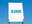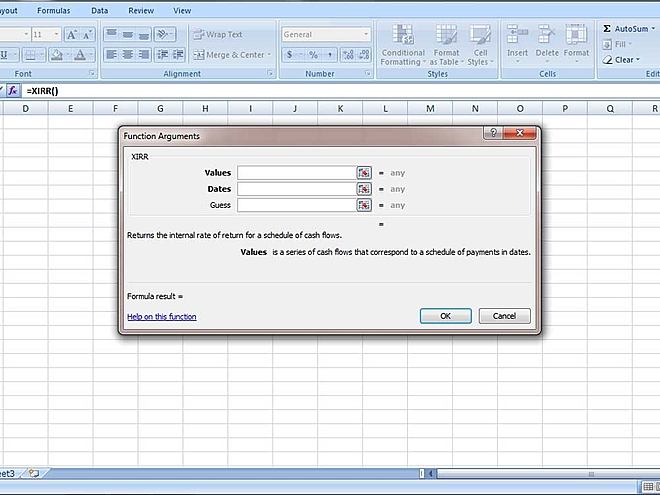# XIRR - A simple explanation

Let's understand what is XIRR and how it is calculatedThe meaning of XIRR or the Extended Internal Rate of Return in the context of mutual funds is the calculation of return on investments where numerous transactions occur at various periods. To understand XIRR, we need to first understand CAGR (Compound Annual Growth Rate). XIRR is nothing but the aggregation of multiple CAGRs. The percentage return shown in the last column of the overview section in My Investments is calculated by the XIRR method.

Let's take a simple example to understand the CAGR. If you invest Rs 10,000 today and it becomes Rs 15,000 in two years, you've made an annual compounded return of 22.47 per cent. This is easy to compute since it is one single investment and at one specific date.

But in the case of an SIP, we would have made not one but several investments. It's also spread across different time frames. To calculate a simple total return would become difficult.

So we use the XIRR formula to calculate the overall CAGR of all the investments. XIRR is used to calculate the internal rate of return for a cash flow for a series of investments done at different periods. So it is nothing but the rate of return earned for varying amounts of money invested for different periods.

Let's take another example - suppose you invested Rs 20,000 in ABC Fund via SIP every month for six months totalling to Rs 1.20 lakh. Since there are six different installments, it's difficult to calculate the total return. Using the XIRR formula, we can easily find out that we earned a total return of 25.17 per cent.

You can use MS Excel to find out XIRR on your own. Just use the XIRR formula and input the 'Values' and 'Dates'.In the values field, add the series of installments with the final return amount. And in the dates field, put the schedule of payments that match the installment.

Piotroski F-score: Rating a company's financial health

C-score: Dodging creative accounting

## Recommended Stories

Should you look at mutual fund size (AUM) before investing?

##### 3 min read •By Ravi Banagere

Should a retiree invest in a technology fund?

How to consolidate your mutual fund portfolio?